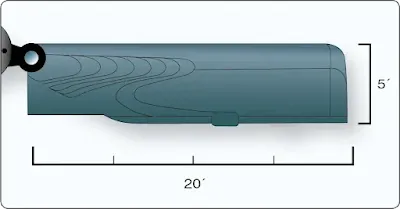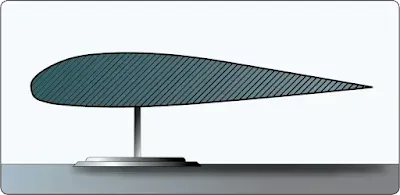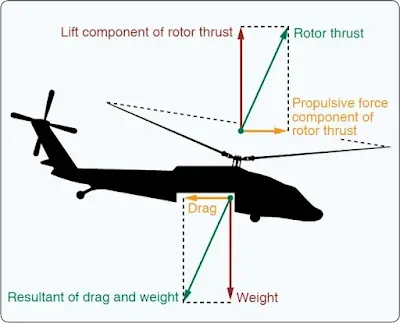# Aerodynamics of Flight

This section presents aerodynamic fundamentals and principles as they apply to helicopters. The content relates to flight operations and performance of normal flight tasks. It covers theory and application of aerodynamics for the pilot, whether in flight training or general flight operations.

Gravity acting on the mass (the amount of matter) of an object creates a force called weight. The rotor blade below weighs 100 lbs. It is 20 feet long (span) and is 1 foot wide (chord). Accordingly, its surface area is 20 square feet. [Figure 1]Figure 1. Area of a blade

The blade is perfectly balanced on a pinpoint stand, as you can see in Figure 2 from looking at it from the end (the airfoil view).Figure 2. Profile of an airfoil

The goal is for the blade to defy gravity and stay exactly where it is when we remove the stand. If we do nothing before removing the stand, the blade will simply fall to the ground. Can we exert a force (a push or pull) opposite gravity that equals the 100 lb. weight of the blade? Yes, for example, electromagnetic force could be used. In helicopters, however, we use aerodynamic force to oppose weight and to maneuver.

Every object in the atmosphere is surrounded by a gas that exerts a static force of 2,116 lb per square foot (a force times a unit area, called pressure) at sea level. However, that pressure is exerted equally all over the blade (top and bottom) and therefore does not create any useful force on the blade. We need only create a difference of a single pound of static pressure differential per square foot of blade surface to have a force equal to the blade’s weight (100 lb of upward pressure opposite 100 lb downward weight).

Total pressure consists of static pressure and, if the air is moving, dynamic pressure (a pressure in the direction of the air movement). As shown in Figure 3, if dynamic pressure is increased the static pressure will decrease. Due to the design of the airfoil, the velocity of the air passing over the upper surface will be greater than that of the lower surface, leading to higher dynamic pressure on the upper surface than on the lower surface. The higher dynamic pressure on the upper surface lowers the static pressure on the upper surface. The static pressure on the bottom will now be greater than the static pressure on the top. The blade will experience an upward force. With just the right amount of air passing over the blade the upward force will equal one pound per square foot. This upward force is equal to, and acts opposite the blade’s weight of 100 lb. So, if we now remove the stand, the blade will defy gravity and remain in its position (ignoring rearward drag for the moment).Figure 3. Four forces acting on a helicopter in forward flight

The force created by air moving over an object (or moving an object through the air) is called aerodynamic force. Aero means air. Dynamic means moving or motion. Accordingly, by moving the air over an airfoil we can change the static pressures on the top and bottom thereby generating a useful force (an aerodynamic force). The portion of the aerodynamic force that is usually measured perpendicular to the air flowing around the airfoil is called lift and is used to oppose weight. Drag is the portion of aerodynamic force that is measured as the resistance created by an object passing through the air (or having the air passed over it). Drag acts in a streamwise direction with the wind passing over the airfoil and retards forward movement.